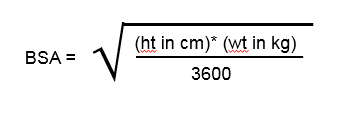## Developing an experiment to test your three hypotheses

You now have three hypotheses about using three easily-measured aspects of an individual’s body to predict how large or small their total lung capacity will be.

Since each of your hypotheses should state that the characteristic it suggests you measure is better at correlating with total lung capacity than any of the others, only one of your hypotheses can be correct about this aspect of the hypotheses. When it comes to the hypotheses that each correlates “well” with total lung capacity, all three might be correct, or none, or some but not others.

To find out which hypothesis is correct about which aspects of its predictions, we do test the predictions! Here, we can test our hypotheses predictions with some simple experiments. It is your job to design those experiments.

The third hypothesis is that total body surface area of individuals correlate best with their total lung capacities. On its face, this does not appear like something it would be easy to measure. It is equivalent to how much area would be taken up by a person’s skin if it were laid on the floor. However, physiologists have developed a number of equations for calculating an individual’s body surface area from just their weight and height. We will use one of the less complicated ones. It allows you to estimate body surface area, BSA, from just a person’s height and weight.Work in a group of three or four students and spend a few minutes to come up with an experimental plan.

Determine:

1. What you will need to measure in different individuals and how you will measure it in order to test all three hypotheses?
2. What you will calculate?
3. What you will graph?
4. How you will decide whether to accept or reject each hypothesis?

After a few minutes, each group will present its ideas to the class and the instructor will fuse the ideas into one well-designed protocol that everyone will follow. Because you need large data sets to analyze, the entire class will end up pooling its data.

### Lab 3 Exercises 3.3

Once the instructor has fused all the ideas into one protocol for everyone to follow, write down the steps here:

1. What you will measure.

1. What you will calculate

1. What you will graph

1. How you will decide whether to reject or accept each hypothesis